# Pandas Boolean Indexing: How to Use Boolean Indexing

In Boolean Indexing, Boolean Vectors can be used to filter the data. Multiple conditions can be grouped in brackets.

## Pandas Boolean Indexing

Pandas boolean indexing is a standard procedure. We will select the subsets of data based on the actual values in the DataFrame and not on their row/column labels or integer locations. Pandas indexing operators “&” and “|” provide easy access to select values from Pandas data structures across various use cases.

Okay, now for this tutorial, we will use the Jupyter Notebook. Also, we need data to work on this project. You can save the CSV file from the below URL.

Now, open the Jupyter Notebook and import the Pandas Library first.

Write the following code inside the first cell in Jupyter Notebook.

`import pandas as pd`

Run the cell by hitting Ctrl + Enter.

Okay, now we will use the  read_csv()function of the DataFrame data structure in Pandas. So write the following code in the next cell.

```data = pd.read_csv('data.csv', skiprows=4)
data```

So, we have used the read_csv() function and skipped the first four rows and then display the remaining rows.

Run the cell and see the output.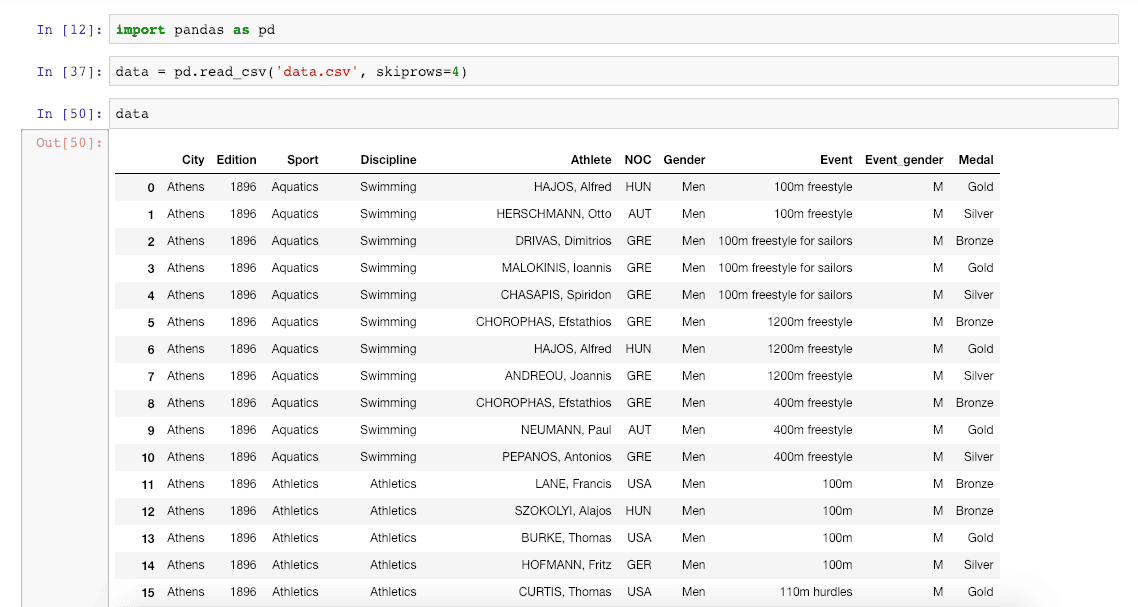Now, let’s use the boolean operator to filter the data.

Let’s take a condition where we will filter out all the data on the athletes who have won the Silver Medal.

Write the following code in the next Jupyter Notebook cell.

`data.Medal == 'Silver'`

So, we are selecting only athletes who have won the Silver Medal.

See the output in Jupyter Notebook.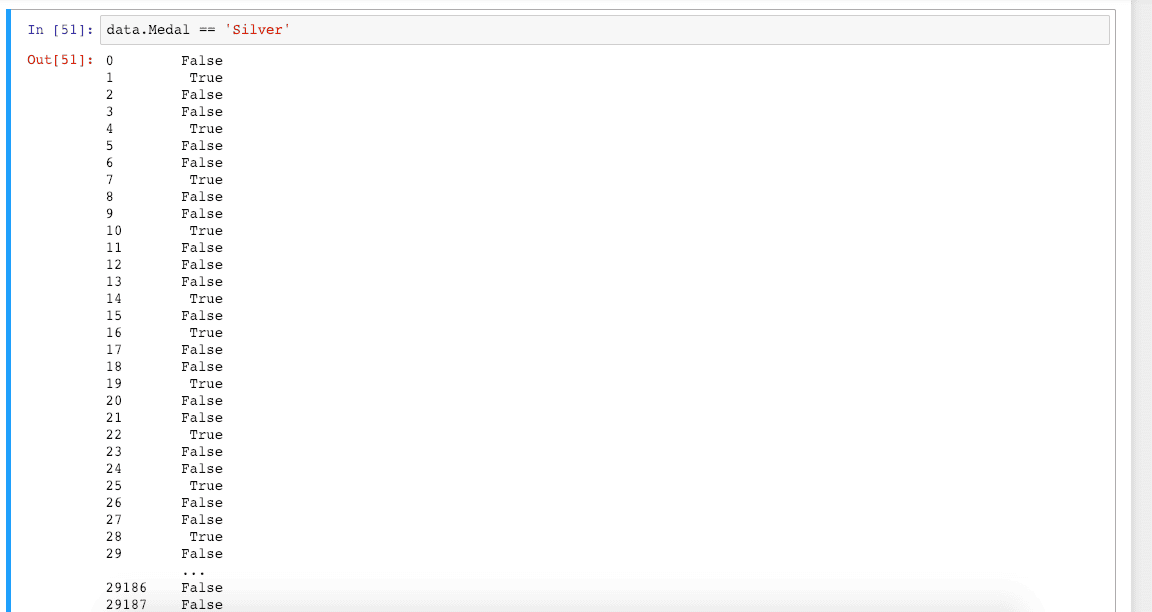Here, we are getting the result dataset in which we get True if an athlete won a Silver medal. Otherwise, we get False.

We can also select the DataFrame based on that, not just the True or False values. So what I mean is that based on the Silver Medal, we can select the athlete name, city, and all the other columns data, a complete DataFrame.

Let’s see how we can display that DataFrame. First, write the following code in the next cell.

`data[data.Medal == 'Silver']`

So, we have wrapped the above code with the data DataFrame and now run that cell, and we can see the complete DataFrame containing only information in which the athletes have won the Silver Medal.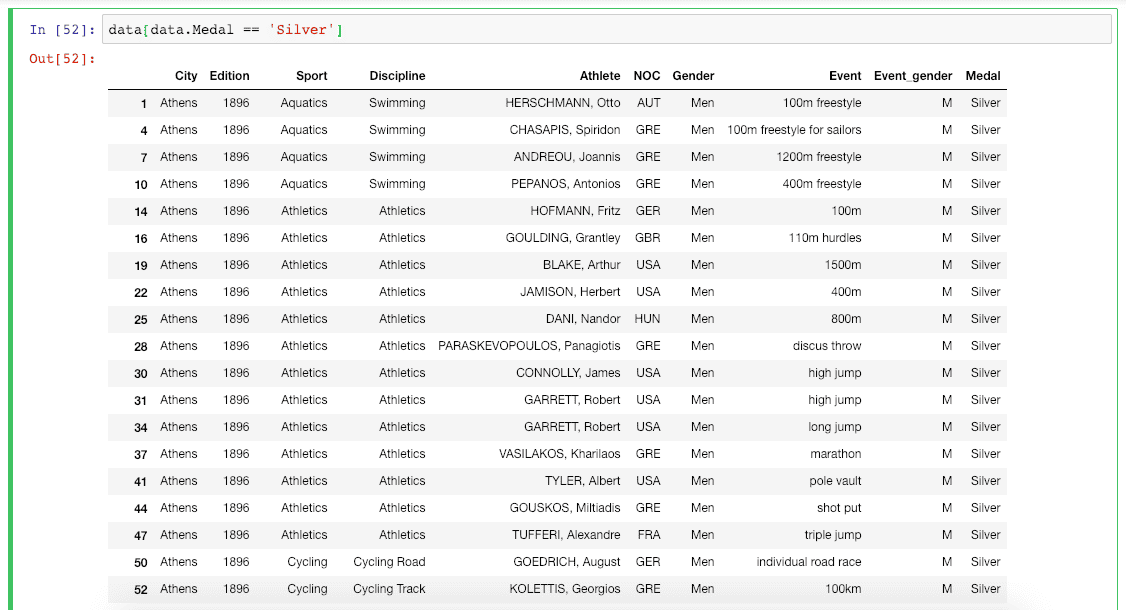## Multiple Conditions in Boolean Indexing

Okay, now we will use the Multiple Conditions to filter the data.

Let’s select all the Men athletes who have won the Silver Medal.

Write the following code in the next cell.

`data[(data.Medal == 'Silver') & (data.Gender == 'Men')]`

In the above code, we have added an And(&) operator to add multiple conditions. Now result in DataFrame contains only the rows which have won the silver medal and all the Men athletes. See the output below.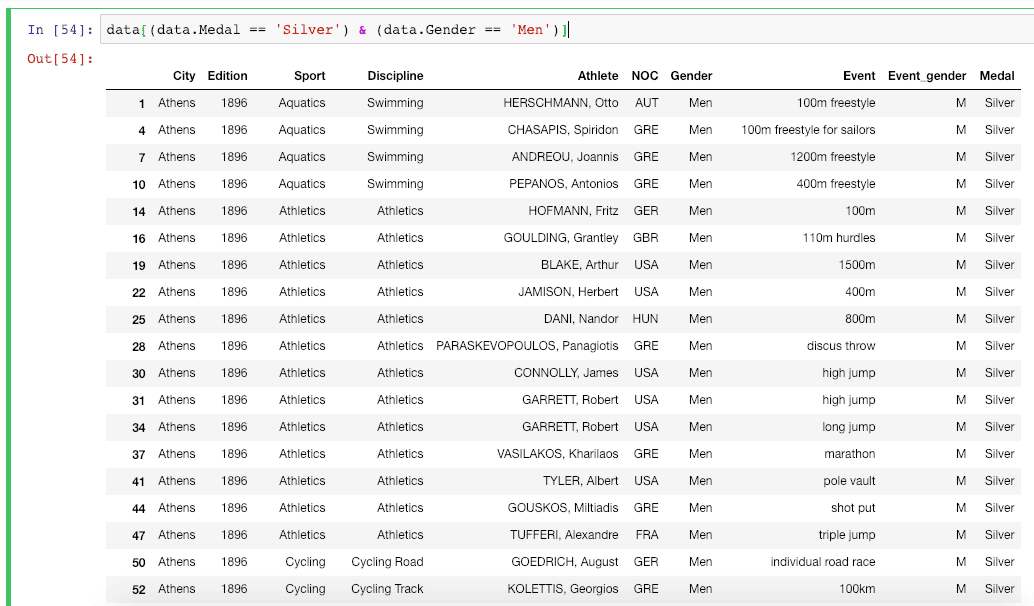## Run Code Without Jupyter Notebook

If you are not using Jupyter Notebook, then you can still run the above code.

I am displaying the demo in Visual Studio Code. Write the following code inside the app.py file. Make sure that you have a data.csv file in the same directory.

```# app.py

import pandas as pd

print(data)
print(data.Medal == 'Silver')
print(data[data.Medal == 'Silver'])
print(data[(data.Medal == 'Silver') & (data.Gender == 'Men')])```

Now, go to the terminal and run the following command.

`python3 app.py`

You can see the output inside the terminal.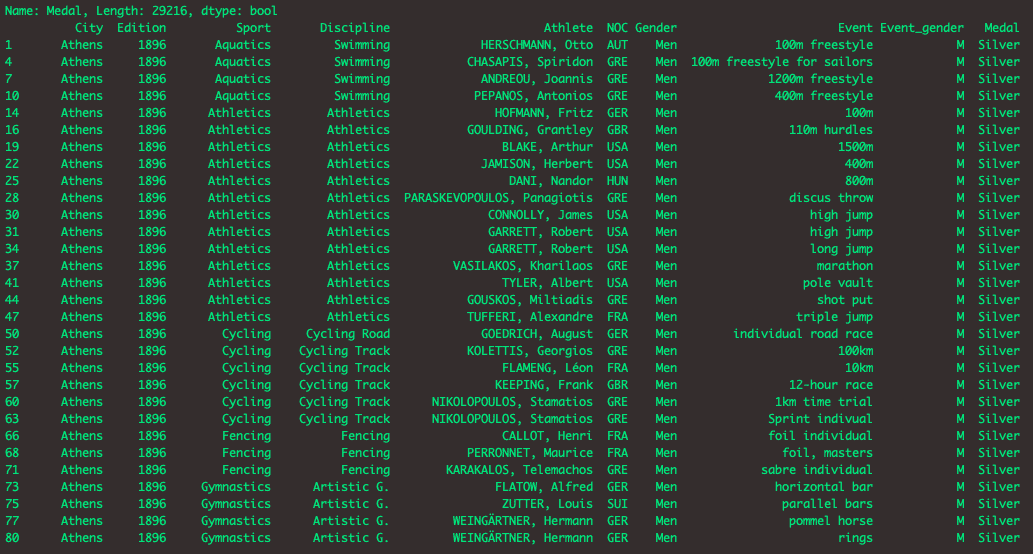That’s it for this tutorial.